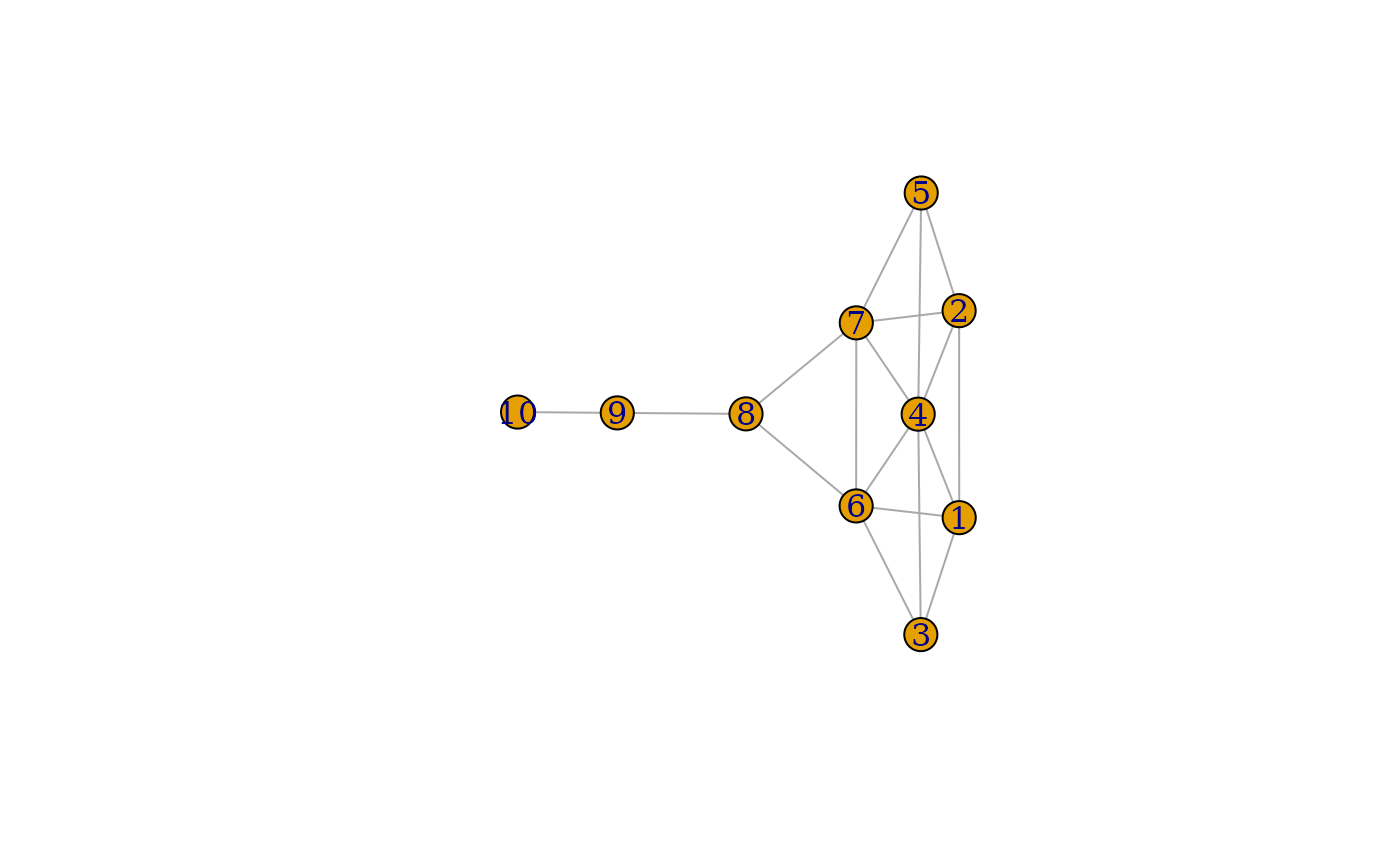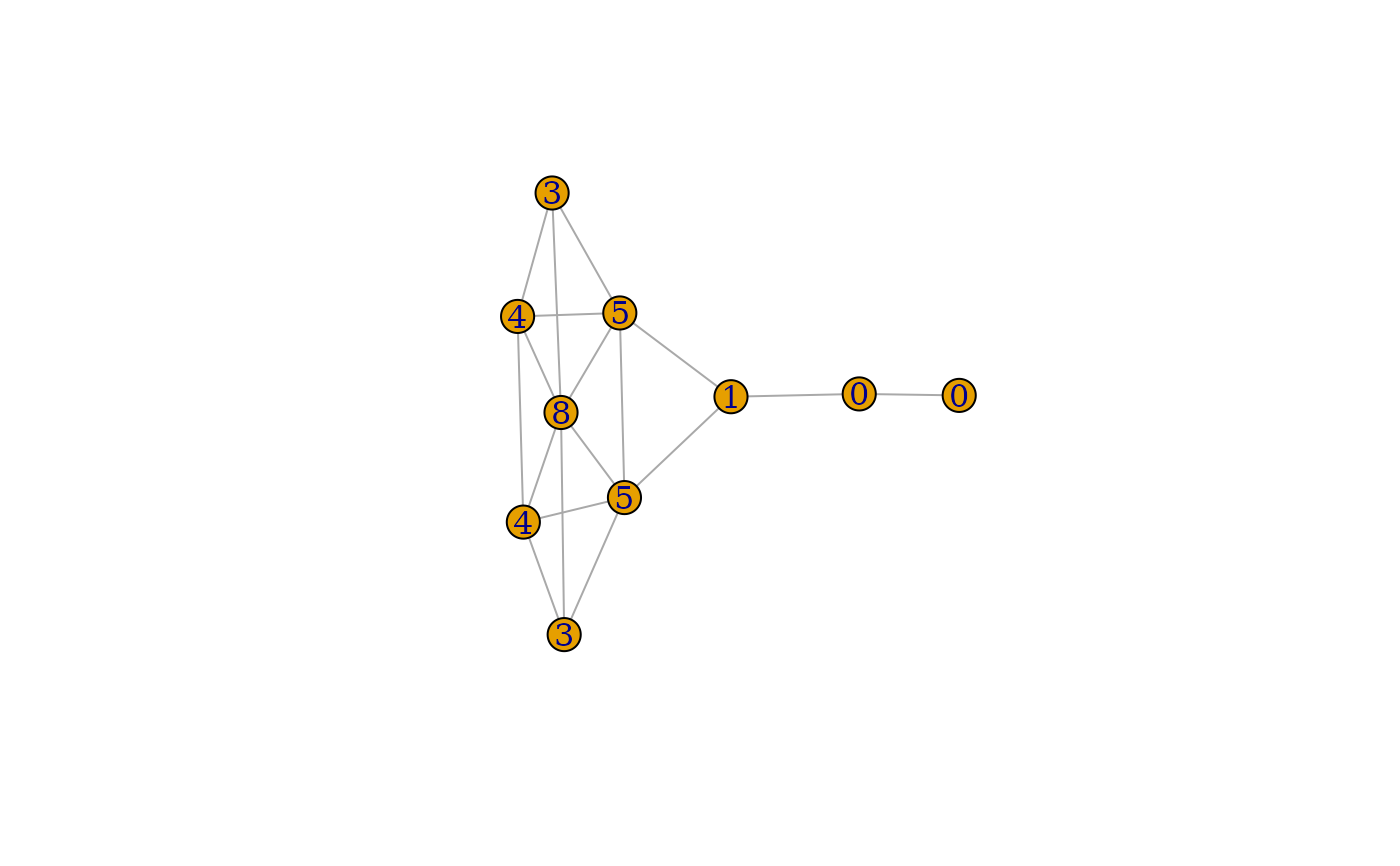Count how many triangles a vertex is part of, in a graph, or just list the triangles of a graph.

## Usage

triangles(graph)

count_triangles(graph, vids = V(graph))

## Arguments

graph

The input graph. It might be directed, but edge directions are ignored.

vids

The vertices to query, all of them by default. This might be a vector of numeric ids, or a character vector of symbolic vertex names for named graphs.

## Value

For triangles() a numeric vector of vertex ids, the first three vertices belong to the first triangle found, etc.

For count_triangles() a numeric vector, the number of triangles for all vertices queried.

## Details

triangles() lists all triangles of a graph. For efficiency, all triangles are returned in a single vector. The first three vertices belong to the first triangle, etc.

count_triangles() counts how many triangles a vertex is part of.

transitivity()

## Author

Gabor Csardi csardi.gabor@gmail.com

## Examples


## A small graph
kite <- make_graph("Krackhardt_Kite")
plot(kite)matrix(triangles(kite), nrow = 3)
#>      [,1] [,2] [,3] [,4] [,5] [,6] [,7] [,8] [,9] [,10] [,11]
#> [1,]    4    4    4    4    4    4    4    4    6     6     7
#> [2,]    1    1    2    6    6    6    7    7    1     7     2
#> [3,]    2    3    5    1    3    7    2    5    3     8     5

atri <- count_triangles(kite)
plot(kite, vertex.label = atri)## Always true
sum(count_triangles(kite)) == length(triangles(kite))
#>  TRUE

## Should match, local transitivity is the
## number of adjacent triangles divided by the number